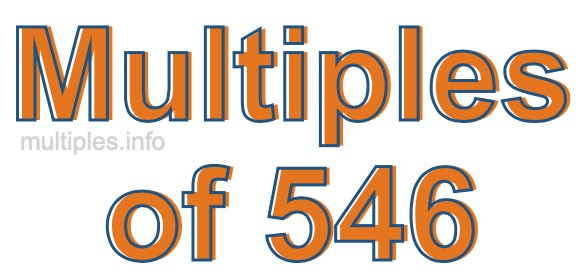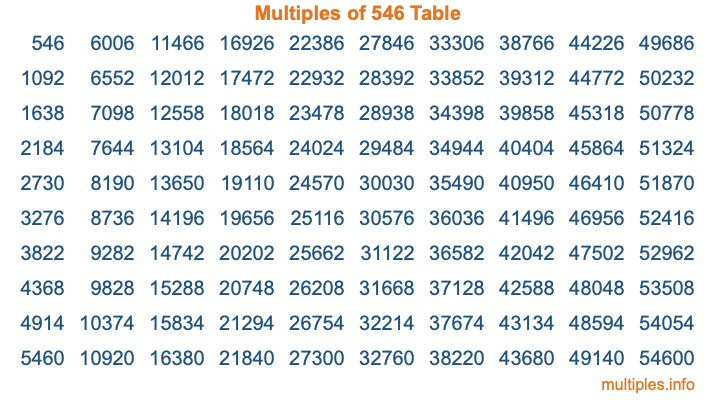Multiples of 546Welcome to the Multiples of 546 page. Here we will first teach you everything you will ever need to know about the multiples of 546, and then give you a study guide summary of everything we taught you to make sure you remember it all. Use this page to look up facts and learn information about the multiples of 546. This page will make you a multiples of five hundred forty-six expert!

Definition of Multiples of 546
Multiples of 546 are all the numbers that when divided by 546 equal an integer. Each of the multiples of 546 are called a multiple. A multiple of 546 is created by multiplying 546 by an integer.

Therefore, to create a list of multiples of 546, you start with 1 multiplied by 546, then 2 multiplied by 546, then 3 multiplied by 546, and so on for as long as you want. Thus, the list of the first five multiples of 546 is 546, 1092, 1638, 2184, and 2730. To see a larger list of multiples of 546, see the printable image of Multiples of 546 further down on this page. We also have a category where you can choose any nth multiple of 546.

Multiples of 546 Checker
The Multiples of 546 Checker below checks to see if any number of your choice is a multiple of 546. In other words, it checks to see if there is any number (integer) that when multiplied by 546 will equal your number. To do that, we divide your number by 546. If the the quotient is an integer, then your number is a multiple of 546.

Is  a multiple of 546?

Least Common Multiple of 546 and ...
A Least Common Multiple (LCM) is the lowest multiple that two or more numbers have in common. This is also called the smallest common multiple or lowest common multiple and is useful to know when you are adding our subtracting fractions. Enter one or more numbers below (546 is already entered) to find the LCM.

Check out our LCM Calculator if you need more details about the Least Common Multiple or if you need the LCM for different numbers for adding and subtraction fractions.

nth Multiple of 546
As we stated above, 546 is the first multiple of 546, 1092 is the second multiple of 546, 1638 is the third multiple of 546, and so on. Enter a number below to find the nth multiple of 546.

th multiple of 546

Multiples of 546 vs Factors of 546
546 is a multiple of 546 and a factor of 546, but that is where the similarities end. All postive multiples of 546 are 546 or greater than 546. All positive factors of 546 are 546 or less than 546.

Below is the beginning list of multiples of 546 and the factors of 546 so you can compare:

Multiples of 546: 546, 1092, 1638, 2184, 2730, etc.

Factors of 546: 1, 2, 3, 6, 7, 13, 14, 21, 26, 39, 42, 78, 91, 182, 273, 546

As you can see, the multiples of 546 are all the numbers that you can divide by 546 to get a whole number. The factors of 546, on the other hand, are all the whole numbers that you can multiply by another whole number to get 546.

It's also interesting to note that if a number (x) is a factor of 546, then 546 will also be a multiple of that number (x).

Multiples of 546 vs Divisors of 546
The divisors of 546 are all the integers that 546 can be divided by evenly. Below is a list of the divisors of 546.

Divisors of 546: 1, 2, 3, 6, 7, 13, 14, 21, 26, 39, 42, 78, 91, 182, 273, 546

The interesting thing to note here is that if you take any multiple of 546 and divide it by a divisor of 546, you will see that the quotient is an integer.

Multiples of 546 Table
Below is an image of the first 100 multiples of 546 in a table. The table is in chronological order, column by column. The first column has the first ten multiples of 546, the second column has the next ten multiples of 546, and so on.The Multiples of 546 Table is also referred to as the 546 Times Table or Times Table of 546. You are welcome to print out our table for your studies.

Negative Multiples of 546
Although not often discussed or needed in math, it is worth mentioning that you can make a list of negative multiples of 546 by multiplying 546 by -1, then by -2, then by -3, and so on, to get the following list of negative multiples of 546:

-546, -1092, -1638, -2184, -2730, etc.

Multiples of 546 Summary
Below is a summary of important Multiples of 546 facts that we have discussed on this page. To retain the knowledge on this page, we recommend that you read through the summary and explain to yourself or a study partner why they hold true.

There are an infinite number of multiples of 546.

A multiple of 546 divided by 546 will equal a whole number.

546 divided by a factor of 546 equals a divisor of 546.

The nth multiple of 546 is n times 546.

The largest factor of 546 is equal to the first positive multiple of 546.

546 is a multiple of every factor of 546.

546 is a multiple of 546.

A multiple of 546 divided by a divisor of 546 equals an integer.

546 divided by a divisor of 546 equals a factor of 546.

Any integer times 546 will equal a multiple of 546.

Multiples of a Number
Here you can get the multiples of another number, all with the same attention to detail as we did for multiples of 546 on this page.

Multiples of
Multiples of 547
Did you find our page about multiples of five hundred forty-six educational? Do you want more knowledge? Check out the multiples of the next number on our list!